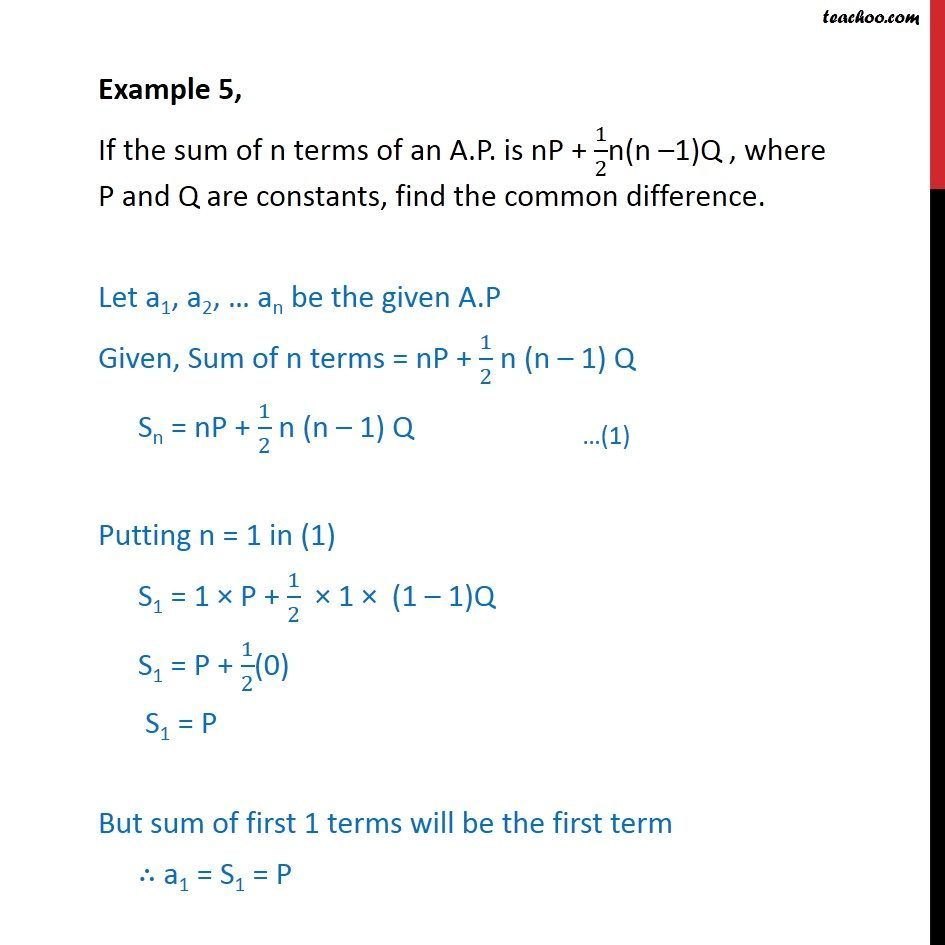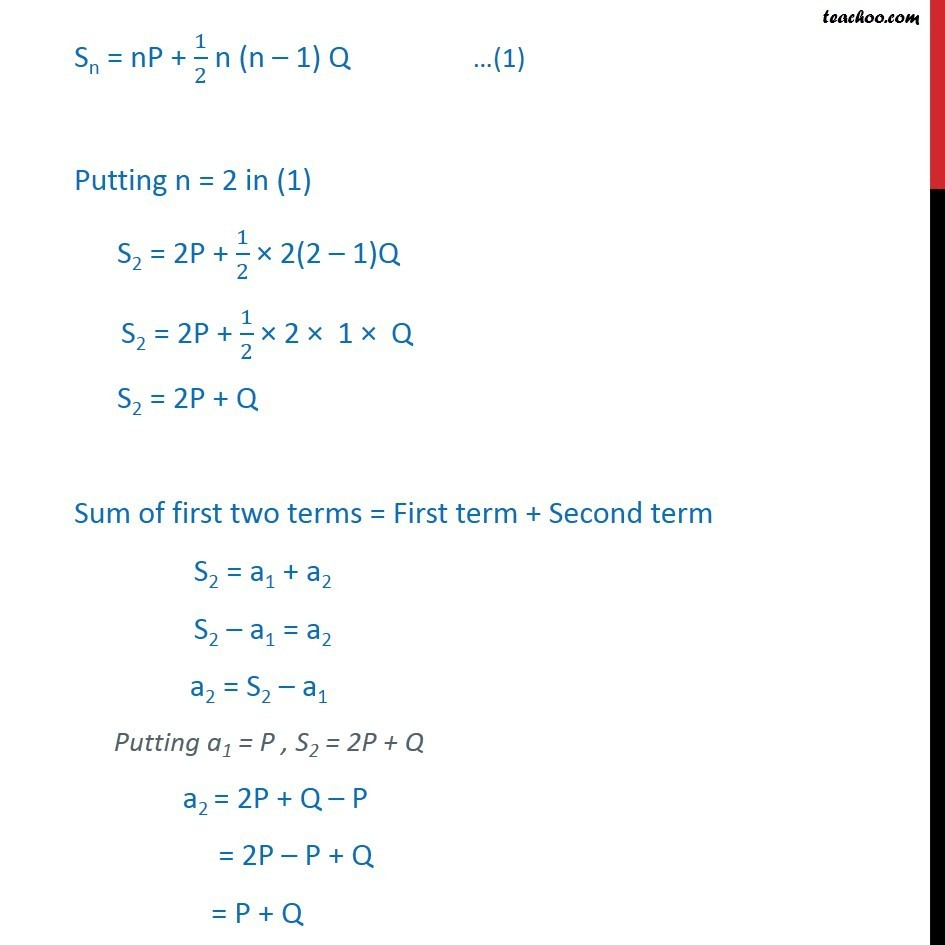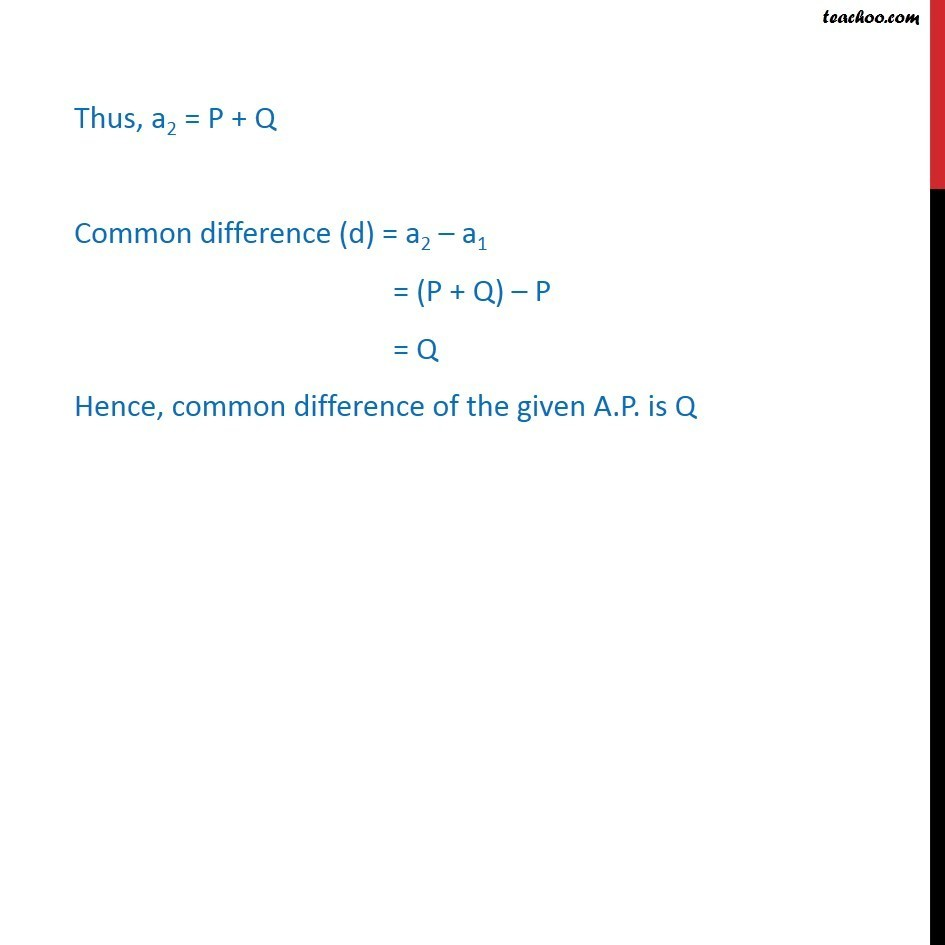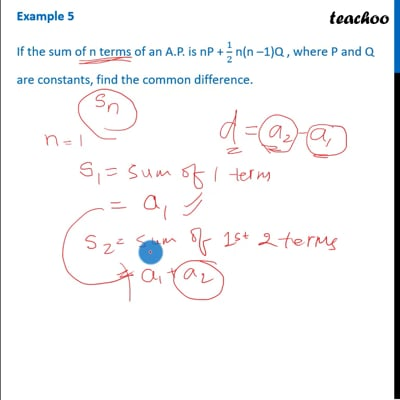Examples

Chapter 9 Class 11 Sequences and Series
Serial order wiseThis video is only available for Teachoo black users

Solve all your doubts with Teachoo Black (new monthly pack available now!)

### Transcript

Example 5, If the sum of n terms of an A.P. is nP + 1/2n(n 1)Q , where P and Q are constants, find the common difference. Let a1, a2, an be the given A.P Given, Sum of n terms = nP + 1/2 n (n 1) Q Sn = nP + 1/2 n (n 1) Q Putting n = 1 in (1) S1 = 1 P + 1/2 1 (1 1)Q S1 = P + 1/2(0) S1 = P But sum of first 1 terms will be the first term a1 = S1 = P Sn = nP + 1/2 n (n 1) Q (1) Putting n = 2 in (1) S2 = 2P + 1/2 2(2 1)Q S2 = 2P + 1/2 2 1 Q S2 = 2P + Q Sum of first two terms = First term + Second term S2 = a1 + a2 S2 a1 = a2 a2 = S2 a1 Putting a1 = P , S2 = 2P + Q a2 = 2P + Q P = 2P P + Q = P + Q Thus, a2 = P + Q Common difference (d) = a2 a1 = (P + Q) P = Q Hence, common difference of the given A.P. is Q